# Preface

Differential equations were invented in the second part of the seventeenth century by I. Newton and G. Leibniz in order to describe some physical phenomena in the appropriate language. The term aequatio differentialis or differential equation was first used by Leibniz in 1676 to denote a relationship between the differentials dx and dy of two variables x and y. In contrast to algebraic equations, differential equations deal with classes of functions. This topic provides a fantastic tool for modeling situations in any field or industry.

Differential equations fall into two very broad categories, called ordinary differential equations (ODE for short) and partial differential equations (PDE). If the unknown function in the equation is a function of only one variable, the equation is called an ordinary differential equation. If the unknown function in the equation depends on more than one independent variable, the equation is called a partial differential equation. This chapter is devoted to some classes of of ordinary differential equation of order one, that is, the equation contains only the first derivative of an unknown function.

Basic terminology

Before beginning to tackle problem formulation and solving differential equations, it is necessary to formulate some basic terminology. First, we reiterate the following notations used to represent a derivative of a function f(x) of one independent variable x:
• In Leibniz's notation, an infinitesimal change in x is denoted by dx, and the derivative of f with respect to x is written $${\text d}f / {\text d} x .$$ The above expression is read as "the derivative of f with respect to x", "df by dx", or "df over dx". The second derivative is denoted as $${\text d}^2 f / {\text d} x^2 .$$
• In Lagrange's notation, the derivative with respect to x of a function f(x) is denoted $$f'(x)$$ (read as "f prime of x") or fx′(x) (read as "f prime x of x"). The second derivative is denoted as $$f'' (x) = {\text d}^2 f / {\text d} x^2 .$$
• In Newton's notation, the derivative with respect to time variable t of a function y(t) is denoted by dot: $$\dot{y} = {\text d}y / {\text d} t .$$ The second derivative is denoted as $$\ddot{y} = {\text d}^2 y / {\text d} t^2 .$$
• Leonhard Euler's notation uses a differential operator suggested by Louis François Antoine Arbogast, denoted as $$\texttt{D} = {\text d} / {\text d} x .$$ The second power of the derivative operator is denoted as $$\texttt{D}^2 = {\text d}^2 / {\text d} x^2 .$$
An ordinary differential equation (ODE) is an equation that contains the derivative or differentials of one dependent variable with respect to one independent variable. If an unknown function depends on several independent variable, we obtain the partial differential equation (PDE).
A differential equation may contain three different types of quantities. The unknown function, for which the equation is to be solved, is called the dependent variable, and when considering ordinary differential equations, the dependent variable is a function of a single independent variable (which we will usually use letters either x or t, for time). In addition to the independent to the independent and dependent variables, a third type of variable, called a parameter, may appear in the equation. A parameter is a quantity that remains fixed in any specification of the problem, but can vary from problem to problem.

A study of differential equations initiated by Gottfried Wilhelm (von) Leibniz in 1676, originated from modeling mechanical problems that are usually written in differentials as

${\text d}y = f(x,y)\,{\text d}x \qquad\mbox{instead of}\qquad \frac{{\text d}y}{{\text d} x} = f(x,y) .$
It is approximated by the equation
$\Delta y = f(x,y)\,\Delta x + \varepsilon \Delta x , \qquad \mbox{where} \qquad \lim_{\Delta x \to 0} \,\varepsilon = 0.$
Here x is the independent variable and y is the dependent variable. Division by Δx yields the equation
$\frac{\Delta y}{\Delta x} = f(x,y) + \varepsilon \qquad \Longrightarrow \qquad \frac{{\text d}y}{{\text d} x} = f(x,y) .$
It should be emphasized that the differential equation follows as an exact mathematical consequence, even though the initial equality is only an approximation.

We utilize the term “differential equations” for the various equations used in this course because they were originally applied to equations formulated in differentials, which deal with incremental changes. The terminology is derived from physics, where they were used to express physical laws such as those of motion, electricity, or magnetism. Differential equations were designed to express these laws such that the physical rules are fixed, yet the same equation could be applied in different contexts. For example, a differential equation of Newton's second law of motion that includes a derivative of velocity with respect to time can be used to find the incremental changes of velocity in a variety of situations. In this course, we adopt the historical convention of calling all equations involving differentials or derivatives, differential equations rather than derivative equations.

ODEs vs PDEs

One important classification is based on whether the unknown function depends on a single independent variable or on several independent variables. This first part of the tutorial deals only with solutions depending on one variable, and they are said to satisfy an ordinary differential equation (abbreviated as ODE). When a differential equation is set for a function depending on several independent variables, we call such equation the partial differential equation (abbreviated as PDE).
Example: Suppose that an object is falling in the atmosphere near sea level. The physical law that governs the motion of objects is Newton's second law, which states that the mass of the object times acceleration is equal to the net force on the object. In mathematical terms, this law is expressed by the equation F = m𝑎, where m is the mass of the object, 𝑎 is its acceleration, and F is the net force exerted on the object. Since the acceleration is the derivative of the velocity v, we can rewrite Newton's second law as
$F = m\,{\text d} v/{\text d}t.$
Next, we consider the forces acting on the falling object: gravity exerts a force equal to the weight of the object, or mg, where g is the acceleration due to gravity, which is approximately 9.8 m/sec² near the earth's surface. There is also a force due to air resistance, or drag force, that is more difficult to model. We may assume that this force is proportional to some power of the velocity. This allows us to write the corresponding equation of motion:
$m\,\frac{{\text d}v}{{\text d}t} = -mg + k_1\,v^{\gamma} \qquad\mbox{or} \qquad \dot{v} = -g + k\,v^{\gamma} .$
■
Example: A typical example of a partial differential equation is the heat conduction equation
$\frac{\partial u}{\partial t} = \kappa\,\frac{\partial^2 u}{\partial x^2}$
and the wave equation
$\frac{\partial^2 u}{\partial t^2} = c^2\,\frac{\partial^2 u}{\partial x^2} .$
We will study these equations in the second part of the tutorial.    ■

Order of differential equations

The order of a differential equation is the order of the highest derivative of the unknown function that appear in the differential equation.
A first-order differential equation is an equation of the form
$F(x,y, y' ) =0 \qquad\mbox{or} \qquad G \left( x, y, {\text d}x, {\text d}y \right) =0,$
where F(x,y,p) is a real-valued function of three variables, and G(x,y,p,q) is a real-valued function of four variables. It is assumed that the derivative y' at least occurs explicitly on the left-hand side of the above equation with F. A solution to $$F(x,y, y' ) =0$$ is a function $$y = \phi (x)$$ so that $$F\left( x,\phi (x) , \phi' (x) \right) \equiv 0$$ for all x in some open interval (𝑎,b). In particular, we require the solution function to be differentiable, and hence continuous on (𝑎,b). A second order differential equation is the equation of the form
$F(x,y, y' , y'' ) =0 ,$
for some continuous function F of four variables.

We will usually deal with differential equations where the derivative is isolated instead of its general form F(x,y,y') = 0. If the function of three variables F(x,y,p) satisfies the conditions (F is continuously differentiable and the partial derivative Fp ≠ 0) of the implicit function theorem, then p can be expressed as a continuous function p = y' = f(x,y), and we can isolate the derivative.

A first order differential equation is said to be in normal form if it is in the following form:
$y' = f(x,y) \qquad\mbox{or} \qquad M(x,y)\,{\text d}x + N(x,y)\,{\text d}y = 0,$
where prime is used to indicate the derivative: $$y' = {\text d} y / {\text d} x ,$$ and dx, dy stand for differentials.
We will also use dots to identify the derivatives with respect to time variable: $$\dot{y} = {\text d} y / {\text d} t , \quad \ddot{y} = {\text d}^2 y/ {\text d} t^2 .$$

An initial value problem for a first-order differential equation consists of the differential equation together with the specification of a value of the solution at a particular point. That is, initial value problems are of the general form:

$F(x,y, y' ) =0 , \qquad y(x_0 ) = y_0 ,$
where $$(x_0 , y_0 )$$ is a given point on the xy-plane.
Example: The following differential equations are of the first order:
$\left( \frac{{\text d}y}{{\text d}x} \right)^3 = y^2 + \frac{{\text d}y}{{\text d}x} \qquad\mbox{and} \qquad \frac{{\text d}y}{{\text d}x} + \sin \left( y(x) \right) = 0.$
Next two equations are of the second and third order, respectively:
$\frac{{\text d}^2 y}{{\text d}x^2} = y^2 + \frac{{\text d}y}{{\text d}x} \qquad\mbox{and} \qquad \frac{{\text d}^3 y}{{\text d}x^3} + \sin \left( y(x) \right) = 0.$
■

Linear ODEs vs nonlinear ODEs

A linear differential equation is a differential equation that is defined by a linear polynomial in the unknown function and its derivatives, that is an equation of the form
$a_0 (x)\,y(x) + a_1 (x)\,y' (x) + \cdots + a_n (x)\,y^{(n)} (x) = r(x)$
where 𝑎0(x), … , 𝑎n(x) and r(x) are arbitrary differentiable functions that do not need to be linear, and $$y', y'', \ldots , y^{(n)}$$ are the successive derivatives of an unknown function y of the variable x.
Example: There are some linear ordinary differential equations of order one and two, respectively:
$x^2 y' + \left( \sin x \right) y = e^x \qquad\mbox{and} \qquad \left( 1 + x^2 \right) y'' - 3x^3 y' + \left( \cos x \right) y = e^x .$
On the other hand, the Riccati equation and the Abel equation are examples of nonlinear equations of the first order:
$y' = x^2 + y^2 \qquad\mbox{and} \qquad y' = x^2 + y^3 .$
The pendulum equation
$\ddot{\theta} + \omega^2 \sin \theta = 0 , \qquad \ddot{\theta} = {\text d}^2 \theta (t) /{\text d} t^2 ,$
is an example of nonlinear second order differential equation.    ■

Formation of differential equations

We will learn shortly that a differential equation usually has infinitely many solutions depending on arbitrary constants. Now we go in opposite direction and give some examples of the elimination of arbitrary constants by the formation of ordinary differential equations. Historically, the first differential equations were derived from the equation $$F(x,y,c_1, c_2 , \ldots , c_n ) =0$$ upon differentiating n times to eliminate constants c1, … ,cn. For example, if we have one constant, the equation can be solved for it: c = ψ(x,y). Then differentiation with respect to x yields the first order differential equation $$\psi_x + \psi_y \,y' =0 .$$ Before 1774 when G. Lagrange introduced the word "general solution," the equation $$F(x,y,c_1, c_2 , \ldots , c_n ) =0$$ was called the primitive for the corresponding differential equation.
Example: Consider a family of ellipses:
$\frac{1}{25} \left( x - a \right)^2 + \frac{1}{4} \left( y - b \right)^2 = r^2 ,$
depending on one parameter R. Differentiating with respect to x and using the chain rule, we obtain
$\frac{1}{25} \left( x - a \right) + \frac{1}{4} \left( y - b \right) y' =0 \qquad \Longrightarrow \qquad y' = - \frac{25}{4} \,\frac{x-a}{y-b} ,$
which is a first order ordinary differential equation.    ■
Example: Consider a family of parabolas
$y^2 = 4a \left( x - h \right)$
that depends on two parameters 𝑎 and h. Differentiating twice, we get
\begin{align*} 2y\,\frac{{\text d}y}{{\text d} x} &= 4a \qquad \Longrightarrow \qquad y\,\frac{{\text d}y}{{\text d} x} = 2a , \\ y\,\frac{{\text d}^2 y}{{\text d} x^2} + \left( \frac{{\text d}y}{{\text d} x} \right)^2 &= 0 , \end{align*}
which is of the second order differential equation.    ■
Example: Consider a trigonometric function
$x(t) = A\,\sin \left( \omega t - \alpha \right)$
that depends on three arbitrary (not necessarily positive) real parameters: A, ω, and α. Differentiating twice, we get
\begin{align*} \dot{x} &= \omega A\,\cos \left( \omega t - \alpha \right) , \\ \ddot{x} &= - \omega^2 A\,\sin \left( \omega t - \alpha \right) = -\omega^2 x(t) \end{align*}
So we get the second order equation of simple harmonic oscillations depending on one parameter: $$\ddot{x} + \omega^2 x = 0 .$$ Of course, this parameter ω can be also eliminated to give the third order differential equation
$\frac{{\text d}}{{\text d}t} \left( \frac{\ddot{x} (t)}{x(t)} \right) = 0 .$
■

Initial Value Problems

A general first order differential equation
$F(x,y, y' ) =0 ,$
where $$y' = {\text d}y/{\text d}x$$ is the derivative of the unknown function y(x), may have a family of solutions depending on a parameter C: $$\phi (x,y ,C ) =0 .$$ This is expected because the derivative operator annihilates any constant. We most likely do not know a formula for the solution ϕ and consider it as a general expression for representing a family of solutions. By specifying particular values for C, we obtain a particular solution to the given differential equation.

There is another approach to knock down a particular solution by specifying the value of unknown function y(x) at particular point. Such condition is called the initial condition: y(x0) = y0, for some specified values x0 and y0. Then the particular value of a constant C can be determined from the equation $$\phi (x_0 ,y_0 ,C ) =0 ,$$ subject that it can be solved.

A differential equation
$F(x,y, y' ) =0$
together with the initial condition $$y\left( x_0 \right) = y_0$$ is called the initial value problem.
Example: Consider the initial value problem for the Riccati equation
$\frac{{\text d}y}{{\text d}x} = x^2 - y^2 , \qquad y(x_0 ) = y_0 ,$
where x0 and y0 are some specified real numbers. If you ask Mathematica to solve a corresponding initial value problem, it will provide you the answer that is hard understand and that requires further analysis and modification.
sol = DSolve[{y'[x] == x^2 - (y[x])^2, y == 1}, y[x], x]
ComplexExpand[sol]
■
If the initial value problem $$y' = f(x,y), \ y(x_0 ) = y_0$$ has a unique solution in some open interval (𝑎 , b) ∋ x0 including the initial point x0 and the solution cannot be extended outside the interval, then this interval (𝑎 , b) is called the validity interval.
We will discuss the validity intervals for autonomous equation later in more details.

Singular Points

It turns out that some points on the plane may not be used to identify the initial condition for a differential equation. Solutions in a neighborhood of some points may exhibit a 'nasty' behavior, such as a cusp or similar feature, which qualify them as exceptional points. At such points, the corresponding initial value problem may have either no solution or many solutions. Therefore, such exceptional points are excluded from consideration and analysis, and we label them with a special term.
If for the initial value-pair (x0, y0) the corresponding solution to the initial value problem
$(a)\ \mbox{ is discontinuous,} \qquad (b)\ \mbox{ is not unique,} \qquad (c)\ \mbox{ does not exist},$
then the pair (x0, y0) is called a singular point of the differential equation. This point can be identified from the equation in normal form. If the slope function f(x,y) of the differential equation $$y' = f(x,y)$$ at some point $$\left( x_0 , y_0 \right)$$ is undefined or is of the following form
$f(x,y) \,\sim \,\frac{0}{0} \qquad\mbox{or}\qquad f(x,y) \,\sim \,\frac{\infty}{\infty} , \quad\mbox{as}\quad x\to x_0 , \ y\to y_0 ,$
then this point is the singular point.

The initial conditions are usually not specified at a singular point because the corresponding initial value problem may have multiple solutions or may have no solution at all.

Example: Consider the differential equation
$\frac{{\text d}y}{{\text d}x} = \frac{y}{x} .$
Its general solution is y = Cx, where C is an arbitrary constant. This solution automatically satisfies the initial condition y(0) = 0 for any value of C. However, if you set the initial condition to be y(0) = 1, the corresponding initial value problem has no solution. We plot the corresponding phase portrait to visualize the singular point.
 LineIntegralConvolutionPlot[{{1, y/x}, {"noise", 500, 500}}, {x, -3, 3}, {y, -3, 3}, ColorFunction -> "BeachColors",) LightingAngle -> 0, LineIntegralConvolutionScale -> 3, Frame -> False]>     Phase portrait for y' = y/x.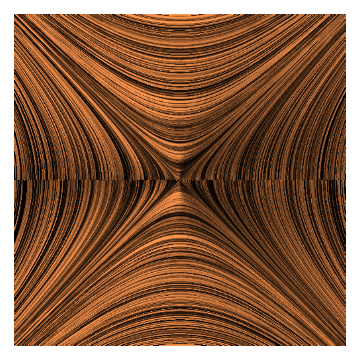Phase portrait for y' = x/y.

On the other hand, a similar differential equation y' = x/y also has a singular point at the origin and we get multiple solutions y = ±x and y ≡ 0 that satisfy the initial condition y(0) = 0.    ■

It is important to note that in a situation where the numerator is nonzero but the denominator is zero, this does not qualify a singular point. This just indicates that the tangent is vertical because dy/dx = ∞, but the geometric interpretation remains meaningful. In this case, we can simply flip the differential equation from dy/dx to dx/dy, moving the zero to the numerator and the nonzero to the denominator. This will give a slope of 0 in the horizontal direction. Thus, there is merit in treating x and y symmetrically, so that the independent variable can be either x or y.

The same conclusion is suggested when differential equations are applied to practical problems. Nature has no cognizance of coordinate systems, which merely provide a framework for the mathematical modeling of an underlying reality. If a problem seems intractable when we insist on a solution y = ϕ(x), but easier when we allow x = ψ(y). It could mean that we have made an inappropriate choice of independent and dependent variables in the initial formulation. These remarks motivate the formulation of the equation in differentials where all variables are treated equally.

Nullclines

A nullcline of the first order differential equation $$y' = f(x,y)$$ is a set of points in the xy- plane so that f(x,y) = 0. Geometrically, these are the points where the solutions go horizontally.
Nullclines are usually not solutions to the differential equation except the particular case when they are constants.
Example: Consider the Riccati equation
$\frac{{\text d}y}{{\text d}x} = x^2 - y^2 .$
It can be easily seen that this equation has two nullclines: $$y = \pm x .$$ As it is seen from the graph, each solution curve intersects nullclines with zero slope.
sol[k_] = DSolve[{y'[x] == x^2 - y[x]^2 , y == k}, y[x], x]
a = Plot[{y[x] /. sol[-1.5], y[x] /. sol[-1], y[x] /. sol, y[x] /. sol, y[x] /. sol}, {x, 0, 2}, PlotStyle -> Thick]
b = Plot[{x, -x}, {x, 0, 2}, PlotStyle -> {{Thick, Magenta}, {Thick, Magenta}}]
Show[a, b]■

The behavior of the integral curves depends strongly on the structure of nullclines around critical points, that is, the multiplicity of nullclines and the sign of slope function.

Equilibrium Solutions

An equilibrium solution (also called a stationary solution or critical point) is a solution to an ordinary differential equation whose derivative is zero everywhere. On a graph an equilibrium solution looks like a horizontal line.
Equilibrium solutions in which solutions that start “near” them move toward the equilibrium solution are called asymptotically stable equilibrium points or asymptotically stable equilibrium solutions. Equilibrium solutions in which solutions that start “near” them move away from the equilibrium solution are called unstable equilibrium points or unstable equilibrium solutions. An equilibrium solution is said to be semi-stable if one side of this equilibrium solution there exists other solutions which approach this equilibrium solution, and on the other side of the equilibrium solution other solutions diverge from this equilibrium solution.

Classifying equilibrium solutions of autonomous differential equations $${\text d}y/{\text d}t = f(y)$$ includes the following steps.

• Draw a vertical line (the phase line) and make tick marks at equilibrium values.
• Between tick marks determine if the slope function f(y) is positive or negative.
• If f(y) is positive, then any solution in this region will be increasing.
• If f(y) is negative, then any solution in this region will be decreasing.
Make conclusion:
• Increasing below and decreasing above     ⇒    asymptotically stable.
• Decreasing below and increasing above     ⇒    unstable.
• Decreasing below and above OR increasing below and above     ⇒     semistable.
Example: Consider the differential equation
$\frac{{\text d}P}{{\text d}t} = P\left( P-1 \right)^2 \left( P - 3 \right) .$
It has three critical points: P = 0, P = 1, and P = 3. By plotting these critical points and evaluating the sign of the derivative, we recognize that P = 0 is an asymptotically stable equilibrium solution, P = 3 is unstable, but P = 1 is a semistable stationary solution.
a = Plot[{0, 1, 3}, {x, 0, 2}, PlotStyle -> Thick];
txt1 = Graphics[ Text[Style["dP/dt > 0 ", FontSize -> 14, Blue], {1.5, -0.4}]];
txt2 = Graphics[ Text[Style["dP/dt < 0 ", FontSize -> 14, Blue], {1.5, 0.5}]];
txt3 = Graphics[ Text[Style["dP/dt < 0 ", FontSize -> 14, Blue], {1.5, 2.0}]];
txt4 = Graphics[ Text[Style["dy/dx > 0 ", FontSize -> 14, Blue], {1.5, 3.5}]];
line = Graphics[{Arrowheads[0.1], Arrow[{{0, -0.6}, {0, 3.5}}]}];
p = Graphics[Text[Style["P", FontSize -> 14, Blue], {0.3, 3.4}]];
t = Graphics[Text[Style["t", FontSize -> 14, Blue], {3.4, 0.2}]];
Show[txt1, txt2, txt3, txt4, a, p,t,line,line2]
StreamPlot[{1, P*(P - 1)^2 *(P - 3)}, {x, 0, 3}, {P, -0.5, 3.5}, VectorPoints -> Fine, StreamColorFunction -> "Rainbow"]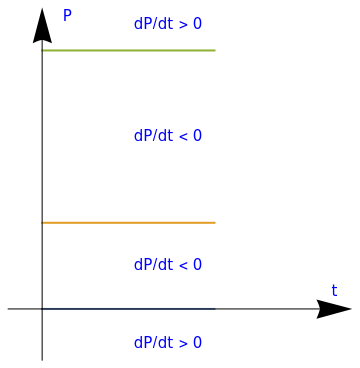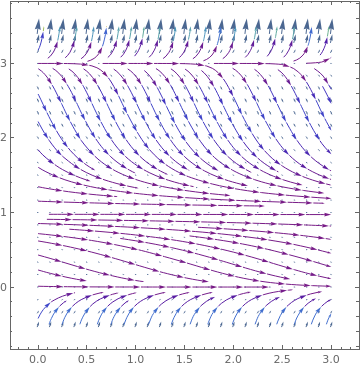■
If for a differential equation $${\text d}y/{\text d}x = f(x,y)$$ there exists an exceptional curve (or curves) that separates two regions of space, each characterized by a specific behavior of solutions, then this curve is called the separatrix for the given differential equation.
Equilibrium solutions can be generalized by including not straight lines.
A curve on the plane is called an asymptotic curve for the differential equation $${\text d}y/{\text d}x = f(x,y) ,$$ if any solution starting in a neighborhood of the curve approaches this curve or departs the curve. Correspondingly, the former is called asymptotically stable asymptotic curve and the latter is called the unstable asymptotic curve.

The next example will show that there exist some curves but not straight lines. In this case it can be seen that solutions approach some curve, but not straight line. However, it is not easy to find such curve, and you need to do some extra work.

Example: Let us consider the differential equation
$\frac{{\text d}y}{{\text d}x} = x^2 - y .$
With Mathematica, we can find its general solution as
solution = DSolve[y'[x] == x^2 - y[x], y[x],x]
{{y[x] -> 2 - 2 x + x^2 + E^-x C}}
$y(x) = 2 - 2\,x + x^2 + C\,e^{-x} ,$
where C is an arbitrary constant. The term containing an exponential function decreases with positive x, so we expect that the other terms will form our equilibrium solution. To confirm this, we use Mathematica and plot:
 sp = StreamPlot[{1, x^2 - y}, {x, -5, 5}, {y, -2, 6}, StreamScale -> {Full, All, 0.04}]]; eq[x_] = 2 - 2 x + x^2; peq = Plot[eq[x], {x, -1.2, 3.2}, PlotStyle -> {Thick, Red}, PlotRange -> {{-1.3, 3.3}, {-1.5, 6}}]; Show[peq, sp]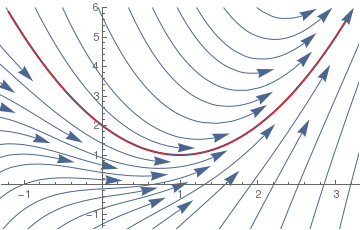Therefore we see that all solutions approach the equilibrium asymptotic solution $$\phi (x) = 2 - 2 x + x^2 ,$$ which is plotted in red.

However, another similar differential equation

$\frac{{\text d}y}{{\text d}x} = x^2 + y$
has the general solution
$y(x) = C\,e^x -2-2\,x-x^2 .$
The asymptotic curve $$\phi (x) = -2 - 2 x - x^2$$ (shown in red) will be unstable.
phi[x_] = -2 - 2 x - x^2
sp = StreamPlot[{1, x^2 + y}, {x, -5, 4.5}, {y, -6, 2}, StreamScale -> {Full, All, 0.04}];
peq = Plot[phi[x], {x, -3.2, 3.2}, PlotStyle -> {Thick, Red}, PlotRange -> {{-3.3, 3.3}, {-5, 2}}];
Show[peq, sp]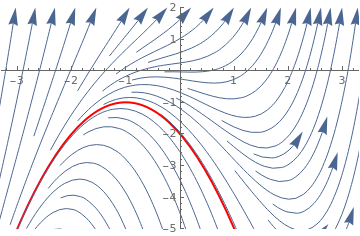■

Solving First Order ODEs

The Wolfram Language function DSolve finds symbolic solutions to differential equations. The Wolfram Language function NDSolve, on the other hand, is a general numerical differential equation solver. DSolve can handle the following types of equations:
• Ordinary Differential Equations (ODEs), in which there are two or more independent variables and one dependent variable. Finding exact symbolic solutions of ODEs is a difficult problem, but DSolve can solve many first-order ODEs and a limited number of the second-order ODEs found in standard reference books.
• Partial Differential Equations (PDEs), in which there are two or more independent variables and one dependent variable. Finding exact symbolic solutions of PDEs is a difficult problem, but DSolve can solve most first-order PDEs and a limited number of the second-order PDEs found in standard reference books.
• Differential Algebraic Equations (DAEs), in which some members of the system are differential equations and the others are purely algebraic, having no derivatives in them. As with PDEs, it is difficult to find exact solutions to DAEs, but DSolve can solve many examples of such systems that occur in applications. DSolve

Verification

Mathematica can be used to verify that known functions are solutions to different differential equations.

Example. To verify that the function $$y=e^{2\,x}$$ is a solution of the differential equation y' - 2 y=0 type:

Clear[y,x]
y[x_]=Exp[2 x]
Out= E^(2 x)
y'[x]-2 y[x]=0
Out= 0

If you enter this syntax correctly, you should get two outputs. The first output should read $$e^{2\,x} .$$ You should get the second output by plugging this potential solution into the differential equation on the third line, which gives a result of zero.

As another example, consider the exponential function multiplied by a constant, $$y=c\,e^{x^2} .$$ We show that this function is a solution to the differential equation $$y' -2x\, y =0$$ independently of arbitrary constant c:

y[x_]=c Exp[x*x]
Simplify[y'[x]-2 x y[x] ==0]
Out= c E^x^2
Out= True
In this syntax, Exp[ ] is the exponential command. It is necessary to make sure that you use the correct brackets. If you do not use the correct type of brackets then the command will not work. We also define a function of a given variable by placing that variable in brackets next to the function. To take derivatives you use '. This means "y-prime" in this context. When you enter this syntax, you once again get two outputs. The first outputs tells you what the function you are defining as y[x_] is. The second output tells you that this statement is true and cannot be simplified.

Some parts of the syntax of the code may be confusing, so that will be explained here. You may wonder why the variable x[k] and y[k] are used instead of x(k) and y(k). The reason is because the brackets [] resemble the denotation you see. Therefore, x[k] and y[k] in actual form would be xk and yk, respectively. The parenthesis, on the other hand, would resemble the independent variable relevant to the dependent variable in the parenthesis.

# 2.1: Motivating examples

Gives some basic and elementary examples involving differential equations of the first order.

# 2.2: Solutions of ODEs

Gives an introduction for utilizing standard Mathematica commands for solving first order ODEs.

# 2.3: Singular solutions

Provides an introduction for singular solutions that may occur while solving some nonlinear differential equations.

# 2.4: Solving first order ODEs

Gives a further classification of differential equations.

# 2.5: Plotting solutions to ODEs

Demonstrates the capability of Mathematica for visualization of solutions to the first order ODEs.

# 2.6: Phase portrait

A tangent field along with some typical solutions is called the phase portrait for the first order differential equation.

# 2.19: Pursuit

1. Abell, M.L. and Braselton, J.P., Differential Equations with Mathematica, Fourth edition, Boston, Academic Press is an imprint of Elsevier, 2020.
2. Dobrushkin, V.A., Applied Differential Equations: The Primary Course, 2021, CRC Press, Boca Raton, FL.
3. Habbard, J.H., What it means to understand a differential equation, The College Mathematics Journal, 1994, Vol. 25, No. 5, pp. 372--384.
4. Ross, C.C. Differential Equations: An Introduction With Mathematica, Second edition, Springer. New York, 2004.